# Plotting Graphs in Mathematica: An Advanced Tutorial

Knoji reviews products and up-and-coming brands we think you'll love. In certain cases, we may receive a commission from brands mentioned in our guides. Learn more.
Mathematica has a beautiful implementation of graph plotting which is used worldwide by scientists, students, engineers in various fields of their work. Multiple graph plotting, piecewise graph plotting, manipulation of a plot, parametric graph plotting,

Mathematica is one of the most popular software used for mathematical computations all over the world. It has several ways of plotting functions and data. But it takes some effort to get used to its commands for plotting graphs and often users fall into difficulty to plot in the way they want. In this article I am going to discuss some of the commonly occurring issues.

1. Multiple graph plotting

This is the most common situation people face while they are in need of comparing two or more graphs. This is very easy and similar to plotting single graphs. First I will show the general command and then explain how it works.Within the first curly brackets you need to put your functions separated by commas and then in the second curly brackets define the range of plot. See the example below.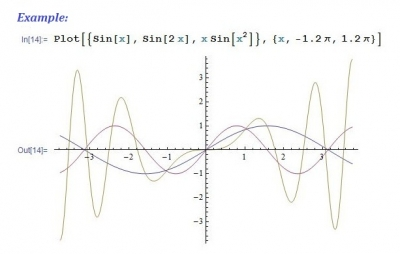2. Piecewise continuous graph plotting

Often we come across functions which are not smooth but piecewise continuous. To plot such functions we need to use a different command.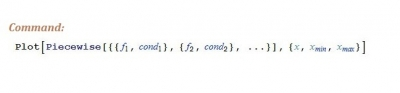In the argument of command “Piecewise” write your functions with the conditions which basically define the range of each individual segment and define the total range of the plot like the previous case. Here is an example.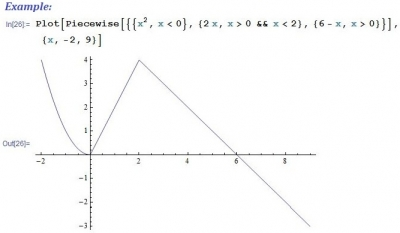3. Manipulate a plot

Very often we need to visualize the effect of a function as a parameter is changed. Mathematica is capable of handling such thing in a beautiful manner.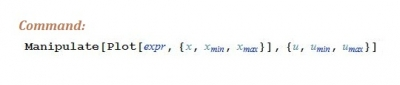Here x defines the plot range and u is the parameter to be manipulated. See the example below.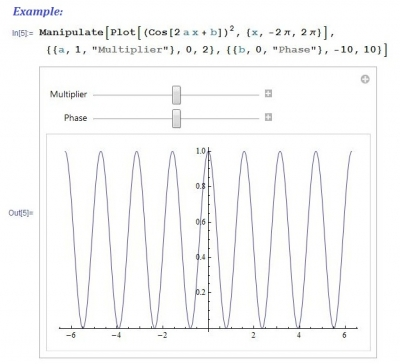The example here is little different from the standard form of command. This shows the flexibility of Mathematica. Here the function is manipulated with respect to two parameters “a” and “b”. Default value of “a” is set to 1 and default value of “b” is set to 0. Parameter “a” is named as “Multiplier” and “b” is named as “Phase”.

4. Parametric plot

Parameterization is one of the beauties of mathematics. In many cases functions take simpler form when represented in parametric form.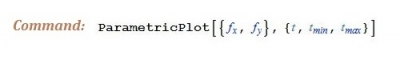Use of this command is very easy as shown in the example.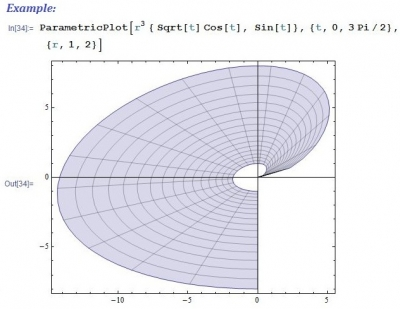5. Plotting graph by importing data from a file(s)

This becomes important when we need to handle a very large amount of data, which is normally output of a program or an experiment, saved in a text file. Plotting graph from a data is little tricky and proper formatting of the data file is required. In our case we shall consider that we have two data files written in the form of two columns of which the first column is the “x” values and the second column is “y” values. Thus our table of data for each of the files will look like follows.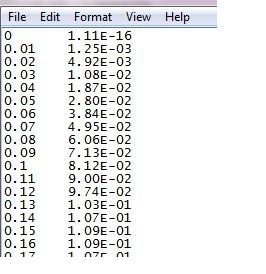We first need to import data from the files and then we will plot them in a single graph. Here are the commands.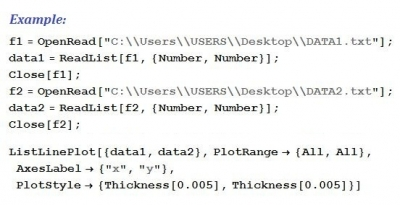Here I have assumed that two data files named as DATA1.txt and DATA2.txt are saved on desktop, otherwise you need to provide correct path of the file. Command “OpenRead” is used to import data. Then those are plotted using command “ListLinePlot”. “PlotStyle” is used to increase thickness of the lines and “AxexLabel” labels the axes. Here is an typical output.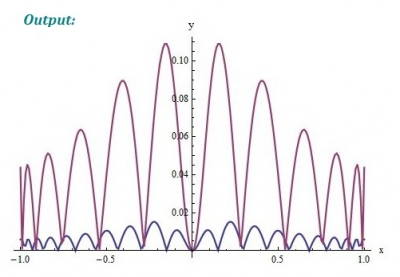Finally I like to mention one good practice; before starting a Mathematica session always reset all the variables by using the following command.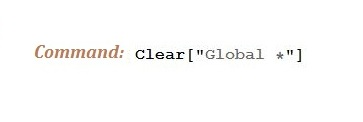This will ensure correctness of the results obtained in Mathematica by erasing the buffer memory.

Hope the article will be helpful in learning plotting in Mathematica.